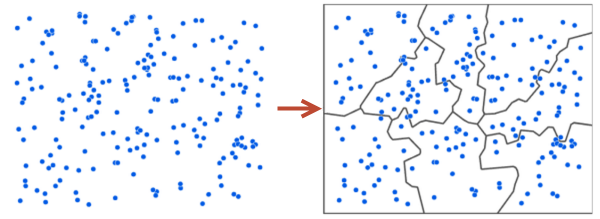# Generate Subset Polygons (Geostatistical Analyst)

## Summary

Generates nonoverlapping subset polygon features from a set of input points. The goal is to divide the points into compact, nonoverlapping subsets, and create polygon regions around each subset of points. The minimum and maximum number of points in each subset can be controlled.

The process of generating subset polygon features begins by connecting all points with a linear curve and cutting this curve into segments. These segments are chosen in order to minimize the total squared distance from the center of the segment to each point in the segment given the minimum and maximum number of points that are allowed to be in each subset. Any overlaps between the segments are then removed, and new segments are created. After several iterations of segmentation and overlap removal, no overlaps will remain, and all points within each segment will be declared part of the same subset. Thiessen polygons are then generated for the input points, and all Thiessen polygons belonging to the same subset are dissolved into a single polygon feature.

## IllustrationPoints (left) are grouped into polygon subsets of a similar size (right).

## Usage

• The primary purpose of this tool is to create polygons that can be used as Subset polygon features in EBK Regression Prediction. The default subset algorithm of EBK Regression Prediction creates subsets that will overlap. However, if you need subsets that do not overlap, this tool can create them.

• This tool is conceptually similar to the tools in the Clustering toolset of the Space Time Pattern Mining toolbox. The main difference is that those tools create point clusters by assigning unique ID values to each member of a cluster, and this tool defines subsets by creating polygon subset regions around the points in each subset. The reason for the difference is that EBK Regression Prediction requires subsets to be defined by polygon regions.

• Consider the number of Input point features when choosing the Minimum number of points per subset and Maximum number of points per subset. If the provided minimum and maximum cannot be honored by the number of input points, an error will be thrown during tool execution. For example, if you have ten points and you ask for a minimum of six points per subset and a maximum of seven points per subset, you will receive an error. This is because there is no way to divide ten points into subsets of size six or seven.

• The default output extent of the Output feature class will be the extent of the Input point features with a 10 percent buffer. This buffering ensures that all input points will be inside the output polygons by default. If an Output extent environment is provided, it will not be buffered.

• Input point features that are in a geographic coordinate system (GCS) will take several times longer to calculate than if the same points are in a projected coordinate system (PCS). This is because data in geographic coordinates will be grouped based on their locations on the earth rather than where they appear on a flat projected map. If your input points are contained in a small area, it is recommended to project your data before using this tool,

## Syntax

`arcpy.ga.GenerateSubsetPolygons(in_point_features, out_feature_class, {min_points_per_subset}, {max_points_per_subset}, {coincident_points})`
 Parameter Explanation Data Type in_point_features The points that will be grouped into subsets. Feature Layer out_feature_class The polygons defining the region of each subset. All points within a single polygon feature are considered part of the same subset. The polygon feature class will contain a field named PointCount that will store the number of points contained in each polygon subset. Feature Class min_points_per_subset(Optional) The minimum number of points that can be grouped into a subset. All subset polygons will contain at least this many points. Long max_points_per_subset(Optional) The maximum number of points that can be grouped into a subset. Each subset will always contain fewer than two times the min_points_per_subset regardless of the maximum number provided. This is because if a subset contains at least twice the minimum number of points, it will always be subdivided into two or more new subsets. Long coincident_points(Optional) Specifies whether coincident points (points that are at the same location) are treated like a single point or as multiple individual points.If you intend to use the subset polygons as Subset polygon features in EBK Regression Prediction, you should maintain consistency between this parameter and your choice for the Coincident points environment in EBK Regression Prediction. If COINCIDENT_ALL is chosen, your Out_feature_class polygons may overlap.COINCIDENT_SINGLE — Coincident points will be treated as a single point in the subsetting. This is the default.COINCIDENT_ALL — Coincident points will be treated as multiple individual points in the subsetting. Boolean

## Code sample

GenerateSubsetPolygons example 1 (Python window)

Group a set of points into polygon subsets.

``arcpy.GenerateSubsetPolygons_ga("myPoints","polygonSubsets",20,30,"COINCIDENT_SINGLE")``
GenerateSubsetPolygons example 2 (stand-alone script)

Group a set of points into polygon subsets.

``````# Name: GenerateSubsetPolygons_Example_02.py
# Description: Groups points into polygon subsets of a similar size.
# Requirements: Geostatistical Analyst Extension
# Author: Esri

# Import system modules
import arcpy

# Set local variables
inPoints = "C:/gapyexamples/input/myPoints.shp"
outFeatureClass = "C:/gapyexamples/output/myPolygons.shp"
minPoints = 50
maxPoints = 75
coincidentPoints = "COINCIDENT_ALL"

# Check out the ArcGIS Geostatistical Analyst extension license
arcpy.CheckOutExtension("GeoStats")

# Execute GenerateSubsetPolygons
arcpy.GenerateSubsetPolygons_ga(inPoints, outFeatureClass, minPoints, maxPoints, coincidentPoints)``````

## Licensing information

• Basic: Requires Geostatistical Analyst
• Standard: Requires Geostatistical Analyst